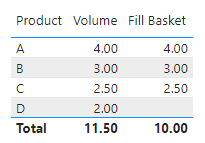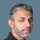cancel
Showing results for
Did you mean:New Member

## Combination of items to fill a basket closest to maximum volume

Hello,

I would like to fill baskets with products to make them as "full" as possible based on volume. For simplicity, lets ignore quantity and other parameters as I account for those elsewhere already.

I have a dataset that looks somewhat like this:

 Product Volume A 4 B 3 C 2,5 D 2

Lets say there is a basket with maximum volume of 10. I need to make a calculation that would output the following resulting in an order of 9,5:

 Product Volume Order A 4 4 B 3 3 C 2,5 2,5 D 2

This should be done in DAX - probably using a calculated table?

Thank you for any suggestions!

1 ACCEPTED SOLUTIONCommunity Support

You can create a Measure as the following.

``````Fill Basket =

VAR total_vol =

CALCULATE ( SUM ( 'Table'[Volume] ), ALL ( 'Table' ) )

VAR accmulate_vol =

CALCULATE (

SUM ( 'Table'[Volume] ),

FILTER ( ALL ( 'Table' ), 'Table'[Volume] >= MAX ( 'Table'[Volume] ) )

)

VAR inter =

CALCULATE (

SUM ( 'Table'[Volume] ),

FILTER ( 'Table', accmulate_vol < basket_size )

)

VAR res =

IF ( HASONEVALUE ( 'Table'[Product] ), inter, basket_size )

RETURN

IF ( total_vol <= basket_size, SUMX ( 'Table', 'Table'[Volume] ), res )``````

The result looks like this:For more details, you can refer the attached pbix file.

Best Regards

Caiyun Zheng

Is that the answer you're looking for? If this post helps, then please consider Accept it as the solution to help the other members find it more quickly.

2 REPLIES 2Community Support

You can create a Measure as the following.

``````Fill Basket =

VAR total_vol =

CALCULATE ( SUM ( 'Table'[Volume] ), ALL ( 'Table' ) )

VAR accmulate_vol =

CALCULATE (

SUM ( 'Table'[Volume] ),

FILTER ( ALL ( 'Table' ), 'Table'[Volume] >= MAX ( 'Table'[Volume] ) )

)

VAR inter =

CALCULATE (

SUM ( 'Table'[Volume] ),

FILTER ( 'Table', accmulate_vol < basket_size )

)

VAR res =

IF ( HASONEVALUE ( 'Table'[Product] ), inter, basket_size )

RETURN

IF ( total_vol <= basket_size, SUMX ( 'Table', 'Table'[Volume] ), res )``````

The result looks like this:For more details, you can refer the attached pbix file.

Best Regards

Caiyun Zheng

Is that the answer you're looking for? If this post helps, then please consider Accept it as the solution to help the other members find it more quickly.Super User

@jan_buzek

I got the idea but your data is not as simple as this sample I believe. Can you share a sample that is much closer to the actual as I think the logic should be applicable at the end.

Did I answer your question? Mark my post as a solution! and hit thumbs up